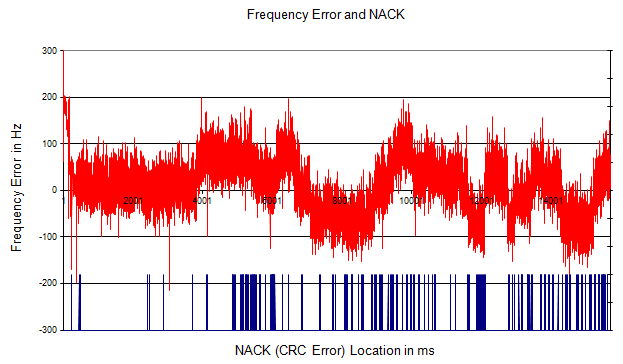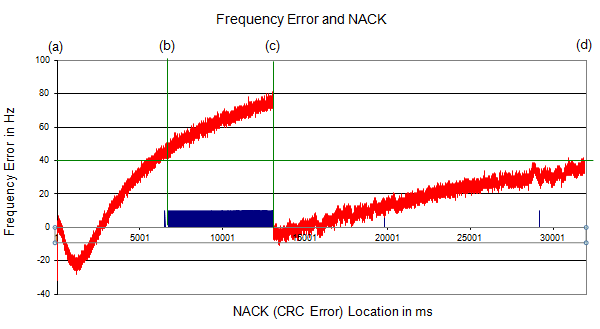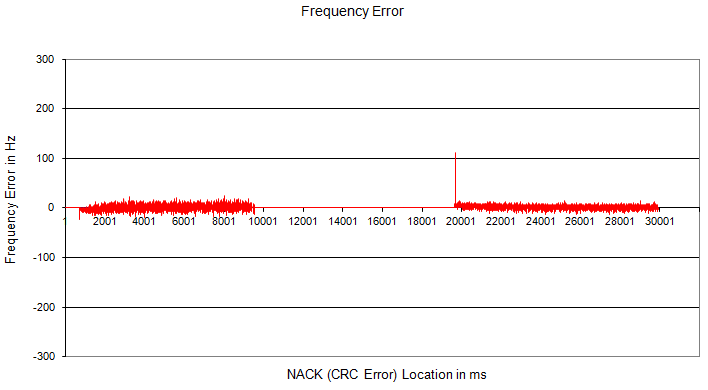LTE Quick Reference Physical Layer Problem Example - Frequency Error   This page shows some correlation between Frequency Error and CRC Error. It is intuitive that you would get more CRC error if you have larger frequency error,but how much frequency error causes how much CRC error is largely physical layer performance implemented by chipset maker.   The acceptable frequency error specified by 3GPP is as follows. (The range is different between eNB and UE).   UE side frequency Error : It is defined in 36.521 6.5.1 Frequency Error. It is specifying the modulated frequency error with reference to received carrier frequency. It is supposed to be +/- 0.1 ppm. For example, if the received carrier frequency is 2.0 Ghz, the frequency error should be within the range of 2.0Ghz +/- 200 Hz. (200 = 2.0 * 10^9 * 0.1 *10^-6)      eNB side frequency Error : It is defined in 36.104 6.5.1 Frequency error. The error should be within +/- 0.05 ppm. For example, if the received carrier frequency is 2.0 Ghz, the frequency error should be within the range of 2.0Ghz +/- 100 Hz.(100 = 2.0 * 10^9 * 0.05 *10^-6)   Frequency Error at baseband : I haven't found any specification for the baseband frequency error. Also, theoretically there shouldn't be any error at the baseband.. but in reality there would be a certain degree of frequency at the baseband mainly because there would be some frequency error with Reference Clock for the baseband. For example, if the frequency error of baseband reference clock is +/- 0.05 ppm, there would be baseband frequency error for 15 Khz (one sub carrier) would be +/- 0.00075 Hz.   Now I will show you a couple of examples of how frequency error would be influencing on data decoding error. This is based on UE side frequency error, but same rule would apply to eNB side as well.   In following plot, the red plot indicate the frequency error for each subframe and the blue bar(line) at the bottom indicate the location of the subframe where CRC error happened. You would notice that the frequency of CRC error goes higher as the range of frequency error goes larger.Following plot  also shows the correlation between frequency error and CRC error. However, this is from a chipset from a different maker. First you would notice how drastically different the overal frequency fluctuation pattern depending on chipset makers.   In this graph, you see that a serious CRC error happens when the frequency error gets higher than a certain point (b) and CRC error disappears when the frequency error gets back to small value (c). (In this chipset, Automatic Frequency Correction was performed at point (c))Following is an ideal case from another chipset maker. As you see, there is negligible amount of frequency error for the whole test span and as a result you don't see any CRC error over the whole period.